You are currently offline. Some features of the site may not work correctly.

# Bell polynomials

Known as: Bell (disambiguation), Complete Bell polynomial, Bell polynomial
In combinatorial mathematics, the Bell polynomials, named in honor of Eric Temple Bell, are used in the study of set partitions. They are related to… Expand
Wikipedia

## Papers overview

Semantic Scholar uses AI to extract papers important to this topic.
2019
2019
• 2019
• Corpus ID: 41819536
In the paper, the authors discuss the Bell polynomials and a sequence of polynomials applied to the theory of differential… Expand
Is this relevant?
2016
2016
• 2016
• Corpus ID: 102492639
We study field diffeomorphisms ϕ(x)→F(ϕ(x))=a0ϕ(x)+a1ϕ2(x)+…=∑j+0∞ajϕj+1,\$\phi (x)\to F(\phi (x))=a_{0}\phi (x)+a_{1}\phi ^{2}(x… Expand
•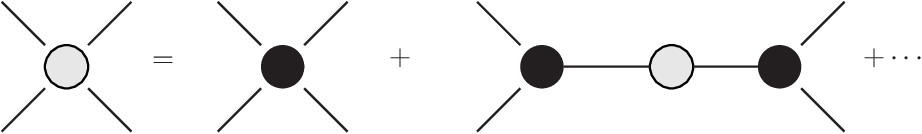•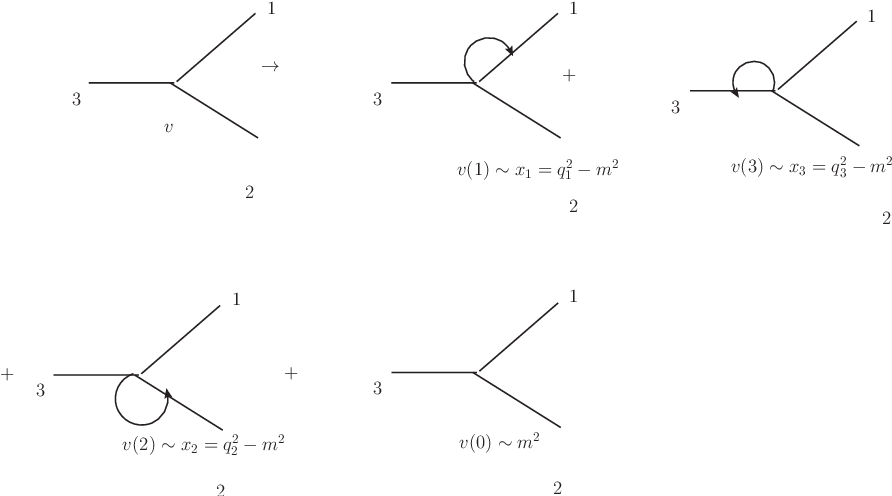•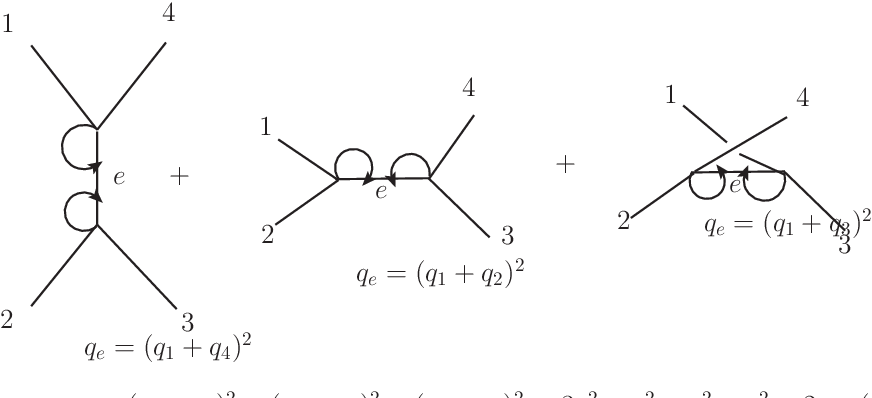•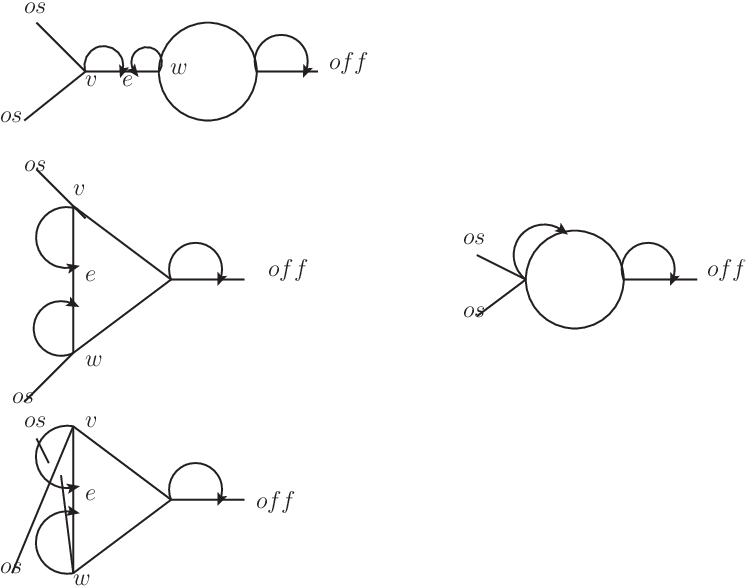•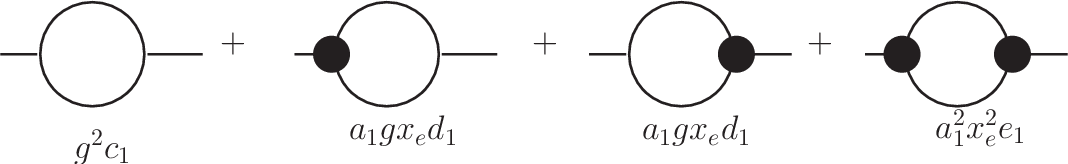Is this relevant?
2014
2014
• 2014
• Corpus ID: 55654080
We introduce how to obtain the bilinear form and the exact periodic wave solutions of a class of (2 + 1)-dimensional nonlinear… Expand
•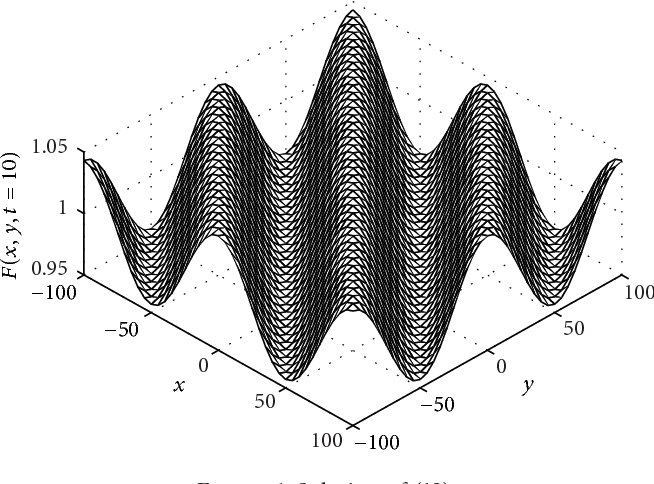Is this relevant?
Highly Cited
2013
Highly Cited
2013
• W. Ma
• 2013
• Corpus ID: 18262310
A class of trilinear differential operators is introduced through a technique of assigning signs to derivatives and used to… Expand
Is this relevant?
Highly Cited
2013
Highly Cited
2013
• W. Ma
• 2013
• Corpus ID: 12934041
A class of bilinear differential operators is introduced through assigning appropriate signs and used to create bilinear… Expand
Is this relevant?
2011
2011
• 2011
• Corpus ID: 18737589
In this paper we use the Euler-Seidel matrix method to obtain some properties of geometric and exponential polynomials and… Expand
Is this relevant?
2009
2009
• Comput. Math. Appl.
• 2009
• Corpus ID: 28232800
The exponential partial Bell polynomials are polynomials in an infinite number of variables x"1,x"2,..., and it is well-known… Expand
Is this relevant?
Highly Cited
2008
Highly Cited
2008
This paper concerns the study of the Bell polynomials and the binomial type sequences. We mainly establish some relations tied to… Expand
Is this relevant?
1996
1996
• Int. J. Algebra Comput.
• 1996
• Corpus ID: 38478552
The recursive definition for the sequence of the Bell polynomials is generalized to noncommutative variables and then explicitly… Expand
Is this relevant?
1987
1987
An introduction to Bell polynomials is given in order to see how they can be used to compute some of the classical counting… Expand
Is this relevant?